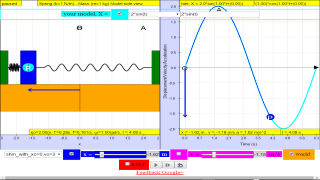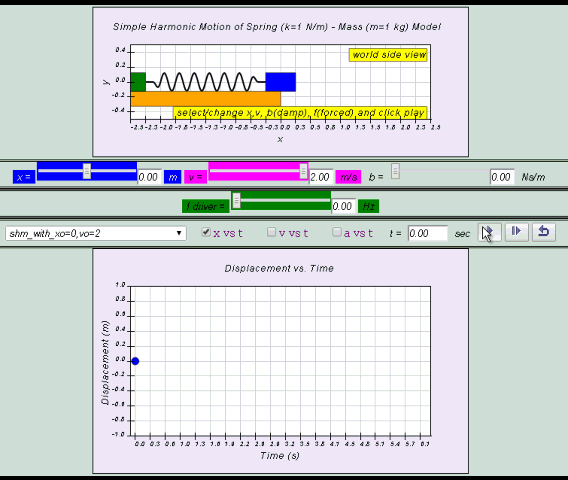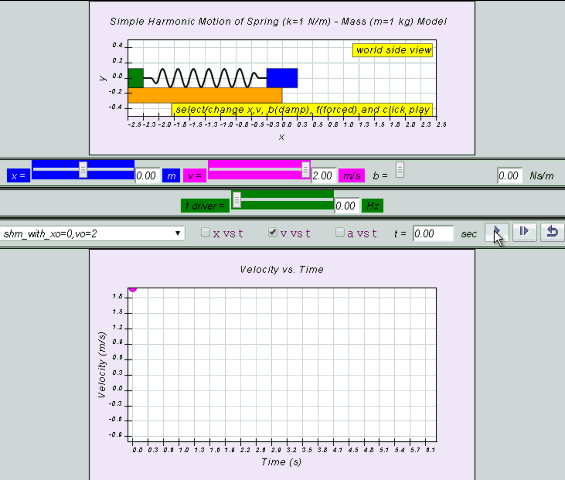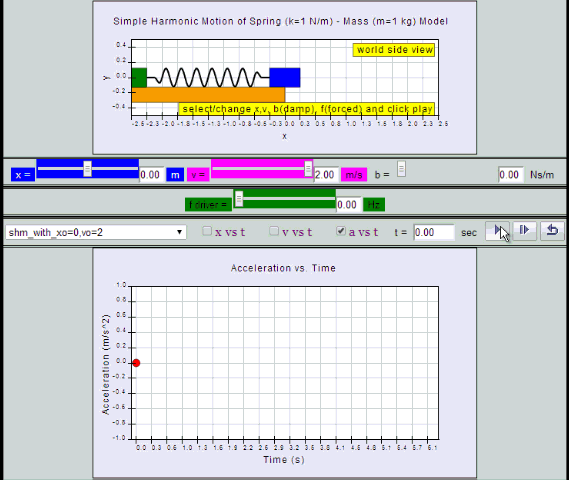SHM08

10.2.2 Special Case (starting from x=0) Solution to the defining equation:LO (e)*

x= x0  sin( ωt )Note:

Equation for v can also be obtained by differentiating x with respect to time t.

v = x0 ω cos (ωt ) = v0 cos (ωt)Note:

Equation for a can also be obtained by differentiating v with respect to time t.

a = - x0 ω2 sin (ωt ) = - a0 sin (ωt)10.2.2.1 Model:

by substitution, suggest if the defining equation a =  - ω2 x is true or false.

10.2.2.2 Suggest there Special Case (starting from x=x0 ) Solution to the defining equation:LO (e) if given

x= x0  cos( ωt )
v = -x0 ω sin (ωt ) = -v0 sin (ωt)
a = -x0 ω2 cos (ωt ) = - a0 cos (ωt)

by substitution, suggest if the defining equation a =  - ω2 x is true or false.

10.2.2.3 Summary:

 Quantity extreme left centre equilibrium extreme right x – x0 0 x0 v 0 + x0ω when v >0 or – x0ω when v <0 which are maximum values 0 a +x0ω2 0 –x0ω2

Translations

Code Language Translator RunSoftware Requirements

SoftwareRequirements

 Android iOS Windows MacOS with best with Chrome Chrome Chrome Chrome support full-screen? Yes. Chrome/Opera No. Firefox/ Samsung Internet Not yet Yes Yes cannot work on some mobile browser that don't understand JavaScript such as..... cannot work on Internet Explorer 9 and below

CreditsThis email address is being protected from spambots. You need JavaScript enabled to view it.

end faq

http://iwant2study.org/lookangejss/02_newtonianmechanics_8oscillations/ejss_model_SHM08/SHM08_Simulation.xhtml

Testimonials (0)

There are no testimonials available for viewing. Login to deploy the article and be the first to submit your review!